# Raster Join

## Description

A common operation for raster data is reprojecting or warping the data to a different CRS with a specific transform. In many use cases, the particulars of the warp operation depend on another set of raster data. Furthermore, the warp is done to put both sets of raster data to a common set of grid to enable manipulation of the datasets together.

In RasterFrames, you can perform a Raster Join on two DataFrames containing raster data. The operation will perform a spatial join based on the CRS and extent data in each DataFrame. By default it is a left join and uses an intersection operator. For each candidate row, all tile columns on the right hand side are warped to match the left hand side’s CRS, extent, and dimensions. Warping relies on GeoTrellis library code. You can specify the resampling method to be applied as one of: nearest_neighbor, bilinear, cubic_convolution, cubic_spline, lanczos, average, mode, median, max, min, or sum. The operation is also an aggregate, with multiple intersecting right-hand side tiles `merge`d into the result. There is no guarantee about the ordering of tiles used to select cell values in the case of overlapping tiles. When using the `raster` DataSource you will automatically get the CRS and extent information needed to do this operation.

## Example Code

Because the raster join is a distributed spatial join, indexing of both DataFrames using the spatial index is crucial for performance.

``````# Southern Mozambique December 29, 2016
spatial_index_partitions=True) \
.withColumnRenamed('proj_raster', 'modis')

spatial_index_partitions=True) \
.withColumnRenamed('proj_raster', 'landsat')

rj = landsat8.raster_join(modis, resampling_method="cubic_convolution")

# Show some non-empty tiles
rj.select('landsat', 'modis', 'crs', 'extent') \
.filter(rf_data_cells('modis') > 0) \
.filter(rf_tile_max('landsat') > 0)
``````

Showing only top 5 rows.

landsat modis crs extent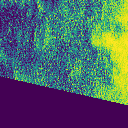[+proj=utm +zone=36 +datum=WGS84 +units=m +no_defs ] [525945.0, -2813925.0, 533625.0, -2806245.0]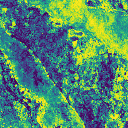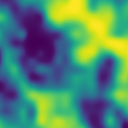[+proj=utm +zone=36 +datum=WGS84 +units=m +no_defs ] [495225.0, -2652645.0, 502905.0, -2644965.0]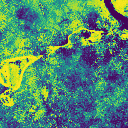[+proj=utm +zone=36 +datum=WGS84 +units=m +no_defs ] [641145.0, -2675685.0, 648825.0, -2668005.0]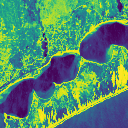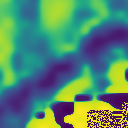[+proj=utm +zone=36 +datum=WGS84 +units=m +no_defs ] [525945.0, -2798565.0, 533625.0, -2790885.0]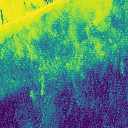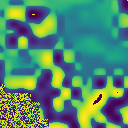[+proj=utm +zone=36 +datum=WGS84 +units=m +no_defs ] [541305.0, -2798565.0, 548985.0, -2790885.0]

The following optional arguments are allowed:

• `left_extent` - the column on the left-hand DataFrame giving the extent of the tile columns
• `left_crs` - the column on the left-hand DataFrame giving the CRS of the tile columns
• `right_extent` - the column on the right-hand DataFrame giving the extent of the tile columns
• `right_crs` - the column on the right-hand DataFrame giving the CRS of the tile columns
• `join_exprs` - a single column expression as would be used in the `on` parameter of `join`
• `resampling_method` - resampling algorithm to use in reprojection of right-hand tile column

Note that the `join_exprs` will override the join behavior described above. By default the expression is equivalent to:

``````st_intersects(
st_geometry(left[left_extent]),
st_reproject(st_geometry(right[right_extent]), right[right_crs], left[left_crs])
)
``````

Resampling method to use can be specified by passing one of the following strings into `resampling_method` parameter. The point resampling methods are: `"nearest_neighbor"`, `"bilinear"`, `"cubic_convolution"`, `"cubic_spline"`, and `"lanczos"`. The aggregating resampling methods are: `"average"`, `"mode"`, `"median"`, `"max"`, “`min`”, or `"sum"`. Note the aggregating methods are intended for downsampling. For example a 0.25 factor and `max` method returns the maximum value in a 4x4 neighborhood.